# Filtering and Fourier Transform

Posted by Ahmet Cecen on October 4, 2016

## Regular 2pt Statistics PCA on Half Vortex### Ahmet Cecen

#### Data Scientist / Materials Informatics# Fourier Space Filtering

Filtering in real space can be represented by the following relationship:

Where $x$ is the filter, and $C$ is a circulant matrix constructed using the input image. For a simple example let us assume the input image and filter are 1D. The matrix $C$ is constructed by shifting the image periodically once at each row. Notice below the 1D image starts with the green pixel and ends with the red, and repeatedly shifted to produce the matrix $C$. The vectors corresponding to these shifts are illustrated to the left. Convince yourself the multiplication in the image below is equivalent to filtering of the 1D image at the first row of the matrix with the yellow filter.Any circulant matrix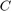can be diagonalized by the Fourier matrix F as: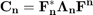Read DFT Matrix and convolution theorem from Wikipedia if you are interested in understanding why this is so.

This fact can be exploited to achieve multiplication of a vector within O(nlogn) time using FFT. The following scheme can be used for this purpose, whereis the first columnand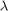is a vector containing the main diagonal of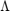: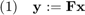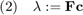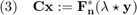where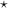is element-wise multiplication. Notice the above algortihm is actually the Convolution Theorem in disguise, where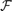is the Fourier Transform operator: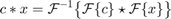The mirror mismatch between convolution and correlation operations can be accounted for by conjugation of the filter in Fourier space.